Induction proof

This page explains how to prove with induction method on the natural number with Rodin tools. In other words, how to prove :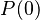$P(0)$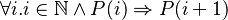$\forall i.i \in \mathbb{N}\land P(i) \Rightarrow P(i+1)$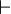$\vdash$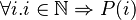$\forall i. i \in \mathbb{N} \Rightarrow P(i)$

The proof key is the following theorem: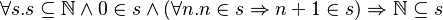$\forall s.s \subseteq \mathbb{N} \land 0 \in s \land (\forall n.n \in s \Rightarrow n+1 \in s)\Rightarrow \mathbb{N} \subseteq s$

The proof of the previous theorem is given by instantiating the key theorem with :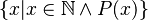$\{x|x\in \mathbb{N} \land P(x)\}$